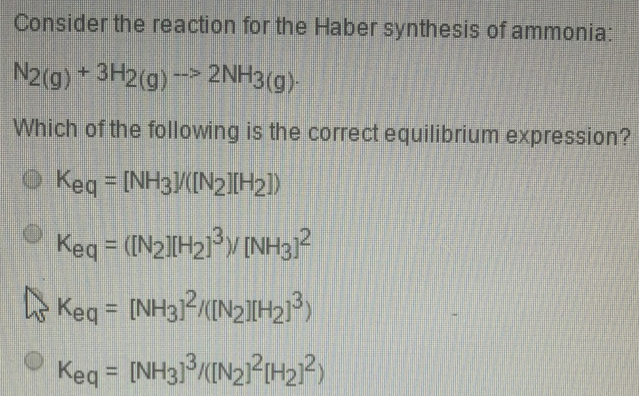# Problem: Consider the reaction for the Haber synthesis of ammonia: N2(g) + 3H2 (g) → 2NH3 (g). Which of the following is the correct equilibrium expression? (i) Keq = [NH3]/[N2][H2]) (ii) Keq = ([N2][H2]3)/[NH3]2 (iii) Keq = [NH3]2/([N2][H2]3) (iv) Keq = [NH3]3/([N2]2[H2]2)

###### FREE Expert Solution
83% (349 ratings)###### Problem Details

Consider the reaction for the Haber synthesis of ammonia:

N2(g) + 3H2 (g) → 2NH3 (g).

Which of the following is the correct equilibrium expression?

(i) Keq = [NH3]/[N2][H2])

(ii) Keq = ([N2][H2]3)/[NH3]2

(iii) Keq = [NH3]2/([N2][H2]3

(iv) Keq = [NH3]3/([N2]2[H2]2)